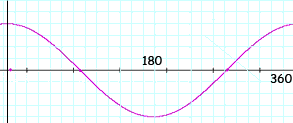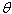Subject: Trig question Name: Christina Who are you: Student I'm have a hard time solving cos2x=1 for exact values between 0<_x <360 We have two responses for you. Hi Christina, I drew a rough sketch of y = cos t for 0 ≤ t ≤ 360 degrees. You can see from the graph that cos t = 1 if t = 0 or t = 360.You also know from the periodic nature of the cosine function that cos t = 1 if t = 0, t = 360, t = 2360 = 720, ... Thus for your function cos 2x = 1 if 2x = 0, 2x = 360, 2x = 2360 = 720, .... That is x = 0, x = 180, x = 360, ... But you only want values of x that satisfy 0 ≤ x < 360 and hence the only values are x = 0 degrees and x = 180 degrees. Penny Hi Christina. Try substituting for 2x. Let= 2x. Then cos= cos 2x. Also, x =/2. So if 0 <= x < 360, then 0 <=/2 < 360, which means 0 <=< 720. Now within the range of 0 <=< 720, for what values ofis cos= 1? You can sketch a cosine curve if you can't answer immediately, or look in your textbook for one. Now that you know all the value(s) of, recall that x =/2, so you can find each corresponding value for x. Hope this helps, Stephen La Rocque. >